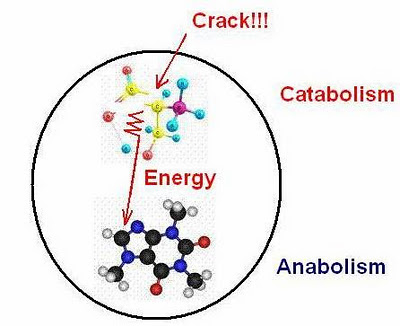## måndag 31 oktober 2011

### Difference Between Emission and Absorption of RadiationIn a sequence of posts on radiative heat transfer and DLR/backradiation I have studied a wave model of the form:
• $U_{tt} - U_{xx} - \gamma U_{ttt} - \delta^2U_{xxt} = f$
where the subindices indicate differentiation with respect to space $x$ and time $t$, and
1. $U_{tt} - U_{xx}$ represents a vibrating string with U displacement
2. $- \gamma U_{ttt}$ is a dissipative term modeling outgoing radiation = emission
3. $- \delta^2U_{xxt}$ is a dissipative modeling internal heating = absorption
4. $f$ is incoming forcing/microwaves,
where $\gamma$ and $\delta^2$ are positive constants connected to dissipative losses as outgoing radiation = emission and internal heating = absorption.

We see emission represented by $-\gamma U_{ttt}$ and absorption by $-\delta^2U_{xxt}$. We now ask:
1. How is the distinction between emission and absorption expressed in this model?
2. Is Helmholtz Reciprocity valid (emission and absorption are reverse processes)?
3. Is Kirchhoff's Radiation Law (emissivity = absorptivity) valid?
Before seeking answers let us recall the basic energy balance between incoming forcing $f$ measured as
• $F = \int f^2(x,t)\, dxdt$
assuming periodicity in space and time and integrating over periods, and (rate of) outgoing radiation = emission $R$ measured by
• $R = \int \gamma U_{tt}^2\, dxdt$,
the oscillator energy $OE$ measured by
• $OE =\frac{1}{2}\int (U_t^2 + U_x^2)\, dxdt$
and (rate of) internal energy = absorption measured by
• $IE = \int \delta^2U_{xt}^2\, dxdt$
• $F = R + IE$
• incoming energy = emission + absorption.
The model has a frequency switch switching from emission to absorption as the frequency increases beyond a certain threshold proportional to temperature in accordance with Wien's displacement law.

Both terms generate dissipative effects when multiplied with $U_t$ as $R = \int \gamma U_{tt}^2\, dxdt$ and $IE = \int \delta^2U_{xt}^2\, dxdt$, but the terms involve different derivatives with $U_{tt}$ acting only in time and $U_{xt}$ acting also in space.

The absorption $U_{xt}^2$ represents a smoothing effect in space, which is irreversible and thus cannot be reversed into emission as reversed absorption.

The emission $U_{tt}^2$ represents a smoothing effect in time, which is irreversible and thus cannot be reversed into absorption as reversed emission.

In other words, in the model both absorption and emission are time irreversible and thus cannot be reversed into each other.

We conclude that the model does not satisfy Helmholtz reciprocity.

Nevertheless, the model satisfies Kirchhoff's law as shown in a previous post.

Conclusion:

The space derivative in $U_{xt}$ models absorption as process of smoothing in space with irreversible transformation of high frequencies in space into low frequencies with a corresponding increase of internal energy as heat energy.

Absorbed high frequencies can with increasing temperature be rebuilt through (resonance in) the wave equation into high frequency emission.

Absorption and emission are not reverse processes, but my be transformed into each other
through (resonance in) the wave equation and the switch.

We may compare absorption with a catabolic process of destroying (space-time) structure and emission with an anabolic process of building structure, with the wave equation as a transformer.

#### 3 kommentarer:

1.Am I interpreting you right in that you model an infinite string of continuously distributed particles in the $x$-direction and that the forcing $f$ represents incoming radiation that makes them vibrate in the lateral direction in relation to $x$. And that $U$ then measures the displacement from the equilibrium string?

And since you insist on strict mathematical rigor I would also like you to do the same in a physical sense. Put in the proper physical constants that apply since the dimensions make no sense at this moment.

As an example, $[U_{xx}] = [\frac{1}{L}] \ne [\frac{T}{L^2}]=[U_{tt}]$ makes no sense unless you work in natural units (which you then should explicitly specify). But in that case it looks like disturbances propagate with the speed of light along the string, is that your intention? I know that mathematicians can be a little bit sloppy with these kind of thing when playing with a toy-model but it is really important that you represent this right when looking at a real situation in the real physical world.

You really should be more explicit in what your model is intended to physically represent.

Sincerely,
Dol

2.The model is a model with periodicity in space and unit speed of light. The precise physical interpretation remains to be done. It is similar to the model used by Planck, but without confusing statistics.

3.Claes wrote:
The precise physical interpretation remains to be done.

What is it that you think that the model represents? Displacements in an electron density?